Fundamentals Of Fluid Mechanics - 8 Edition - Chapter 5.2.2 - Problem 5.77
Register Now

Join StudySoup

Get Full Access to Fundamentals Of Fluid Mechanics - 8 Edition - Chapter 5.2.2 - Problem 5.77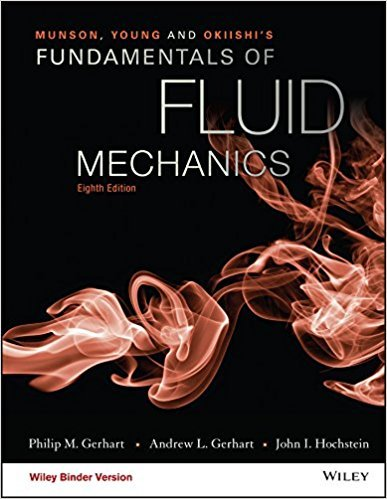9781119080701

# (See The Wide World of Fluids article titled BowThrusters, Section 5.2.2.) The bow

Fundamentals of Fluid Mechanics | 8th Edition

Problem 5.77

(See The Wide World of Fluids article titled BowThrusters, Section 5.2.2.) The bow thruster on the boat shownin Fig. P5.77 is used to turn the boat. The thruster produces a1-m-diameter jet of water with a velocity of 10 m/s. Determinethe force produced by the thruster. Assume that the inlet and outletpressures are zero and that the momentum of the water entering thethruster is negligible.

Accepted Solution
Step-by-Step Solution:

Step 1 of 4

But in this case we have flow of water perpendicular on the boat.Look at picture below.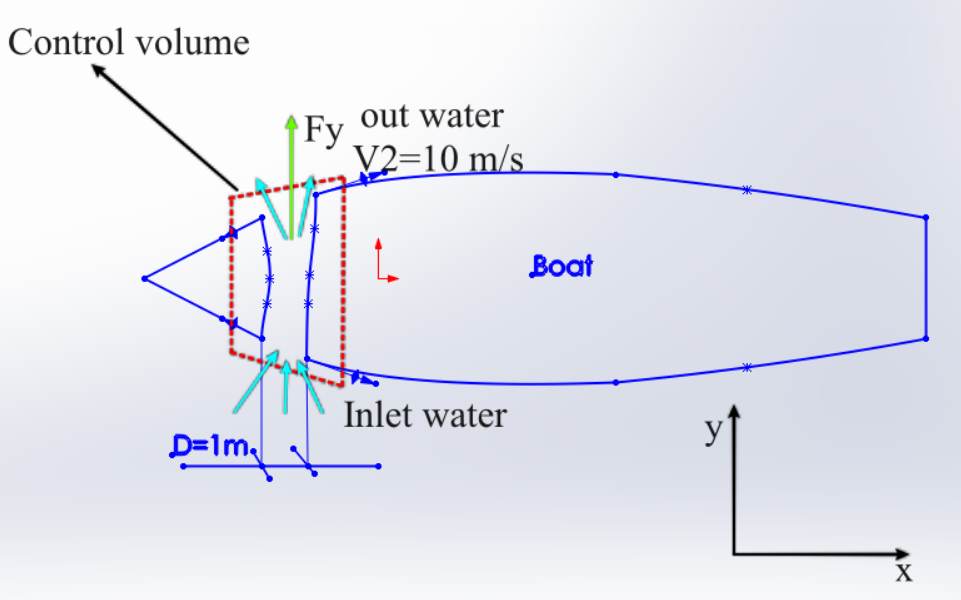Step  2 of 4

We will determine all forces that operate along thedirection. Now we will apply the linear momentum equation to this fixed control volume.Look at picture above.

Where we have:Where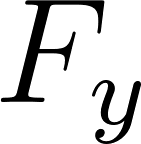is the force produced by  thruster.

Step 3 of 4

If the momentum of the entering water is neglected.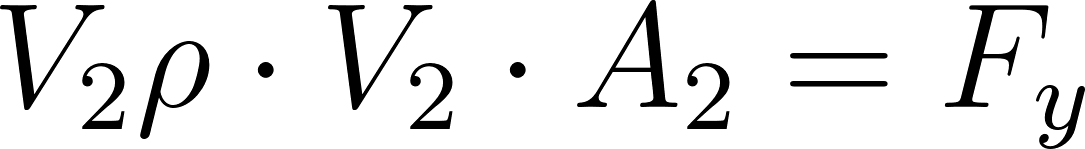Where we have: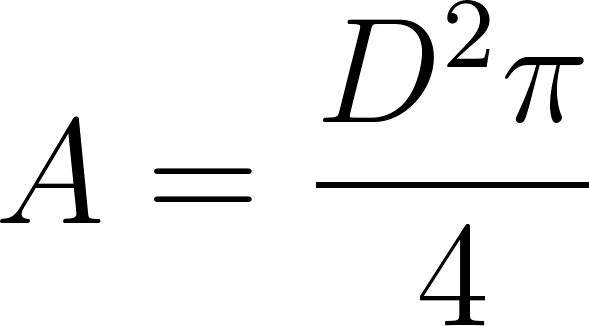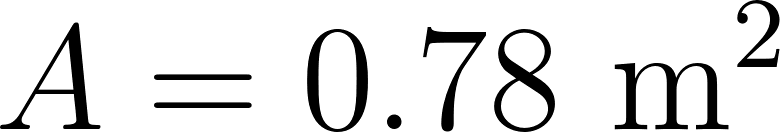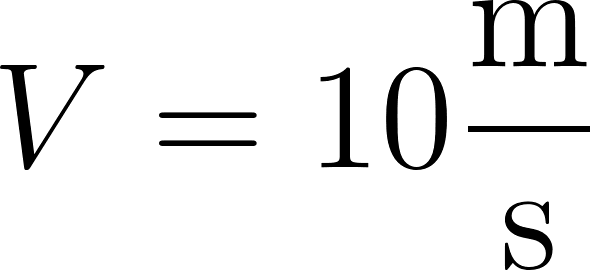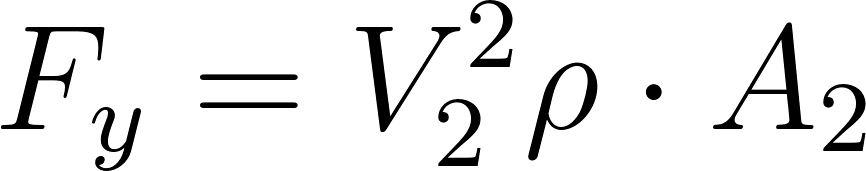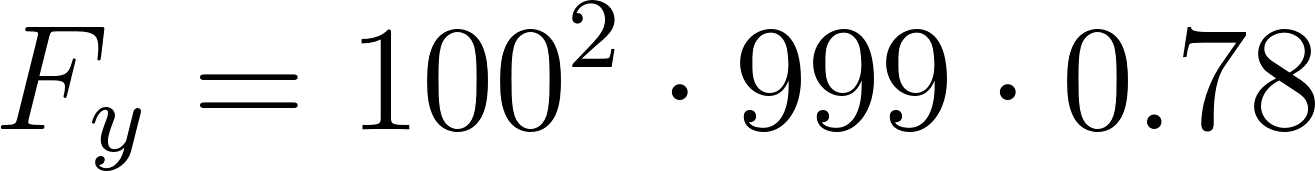Step 4 of 4

Force produced by the thruster is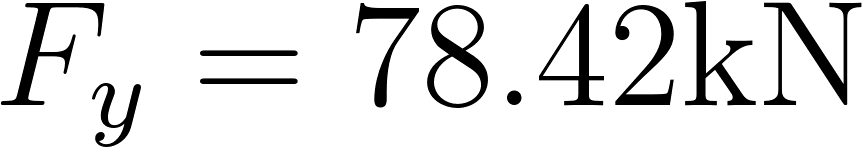###### Chapter 5.2.2, Problem 5.77 is Solved

Step 2 of 4

Step 3 of 4

Unlock Textbook Solution